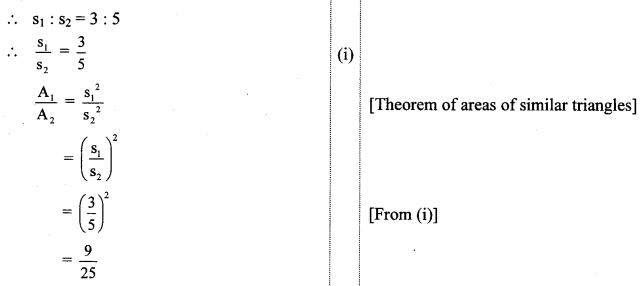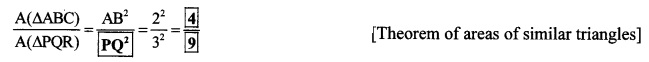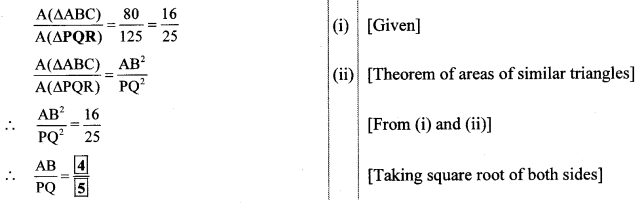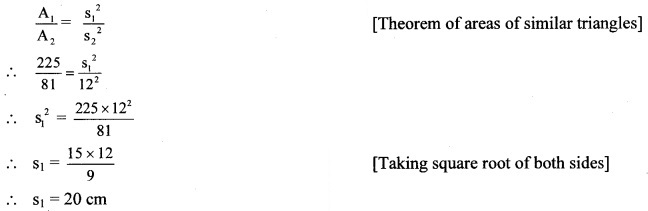# Maharashtra Board 10th Class Maths Part 2 Practice Set 1.4 Solutions Chapter 1 Similarity

Balbharti Maharashtra State Board Class 10 Maths Solutions covers the Practice Set 1.4 Algebra 10th Class Maths Part 2 Answers Solutions Chapter 1 Similarity.

## Practice Set 1.4 Algebra 10th Std Maths Part 2 Answers Chapter 1 Similarity

Question 1.
The ratio of corresponding sides of similar triangles is 3 : 5, then find the ratio of their areas.
Solution:
Let the corresponding sides of similar triangles be S1 and S2.
Let A1 and A2 be their corresponding areas.∴ Ratio of areas of similar triangles = 9 : 25

Question 2.
If ∆ABC ~ ∆PQR and AB : PQ = 2:3, then fill in the blanks.
Solution:Question 3.
If ∆ABC ~ ∆PQR, A(∆ABC) = 80, A(∆PQR) = 125, then fill in the blanks.
Solution:Question 4.
∆LMN ~ ∆PQR, 9 × A(∆PQR) = 16 × A(∆LMN). If QR = 20, then find MN.
Solution:
9 × A(∆PQR) = 16 × A(∆LMN) [Given]
∴ $$\frac{\mathrm{A}(\Delta \mathrm{LMN})}{\mathrm{A}(\Delta \mathrm{PQR})}=\frac{9}{16}$$ (i)
Now, ∆LMN ~ ∆PQR [Given]
∴ $$\frac{\mathrm{A}(\Delta \mathrm{LMN})}{\mathrm{A}(\Delta \mathrm{PQR})}=\frac{\mathrm{MN}^{2}}{\mathrm{QR}^{2}}$$ (ii) [Theorem of areas of similar triangles]
∴ $$\frac{\mathrm{MN}^{2}}{\mathrm{QR}^{2}}=\frac{9}{16}$$ [From (i) and (ii)]
∴ $$\frac{M N}{Q R}=\frac{3}{4}$$ [Taking square root of both sides]
∴ $$\frac{\mathrm{MN}}{20}=\frac{3}{4}$$
∴ MN = $$\frac{20 \times 3}{4}$$
∴ MN = 15 units

Question 5.
Areas of two similar triangles are 225 sq. cm. and 81 sq. cm. If a side of the smaller triangle is 12 cm, then find corresponding side of the bigger triangle.
Solution:
Let the areas of two similar triangles be A1 and A2.
A1 = 225 sq. cm. A2 = 81 sq. cm.
Let the corresponding sides of triangles be S1 and S2 respectively.
S1 = 12 cm∴ The length of the corresponding side of the bigger triangle is 20 cm.

Question 6.
∆ABC and ∆DEF are equilateral triangles. If A(∆ABC): A(∆DEF) = 1:2 and AB = 4, find DE.
Solution:
In ∆ABC and ∆DEF,Question 7.
In the adjoining figure, seg PQ || seg DE, A(∆PQF) = 20 sq. units, PF = 2 DP, then find A (꠸ DPQE) by completing the following activity.
Solution:
A(∆PQF) = 20 sq.units, PF = 2 DP, [Given]
Let us assume DP = x.
∴ PF = 2xScroll to Top